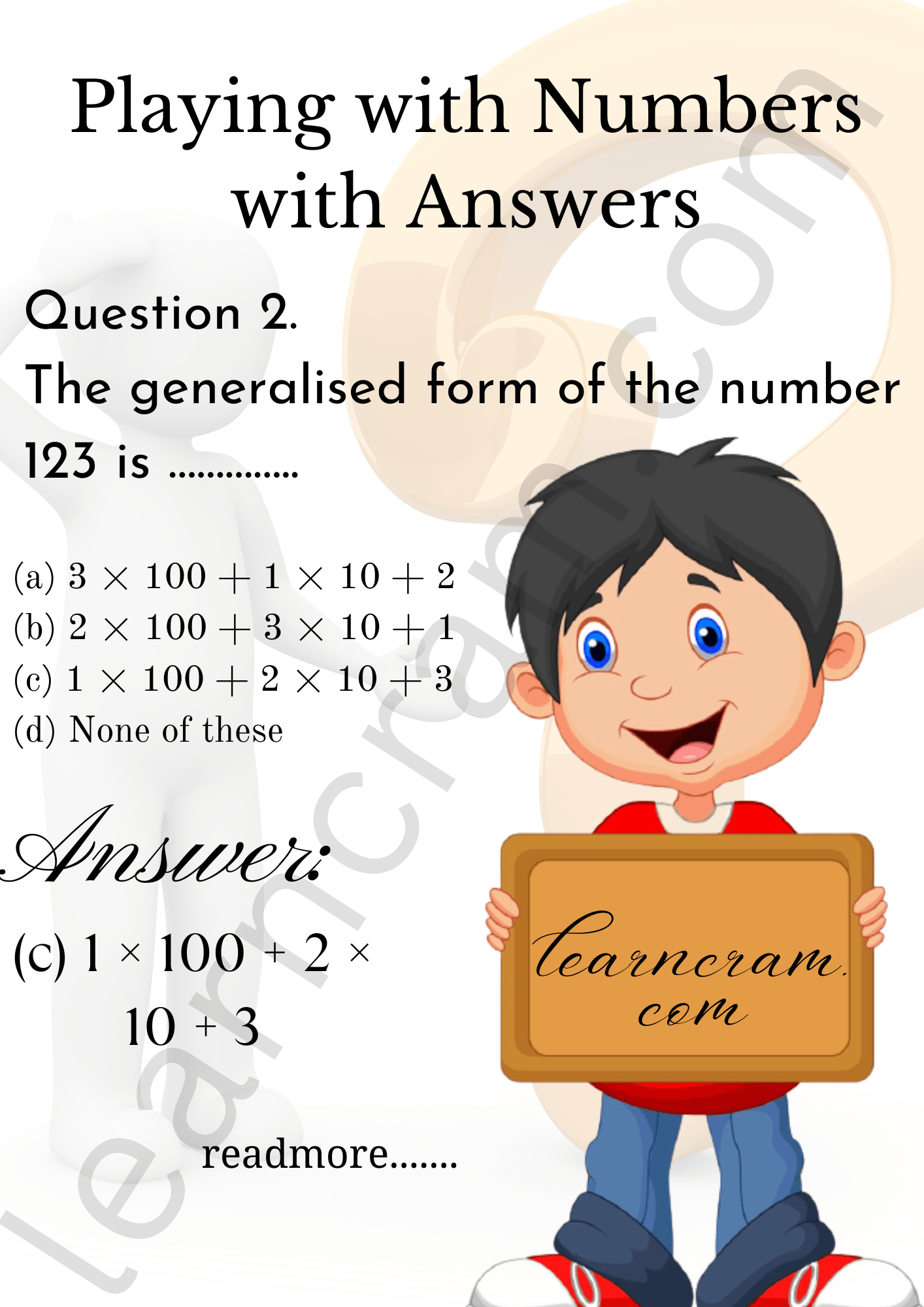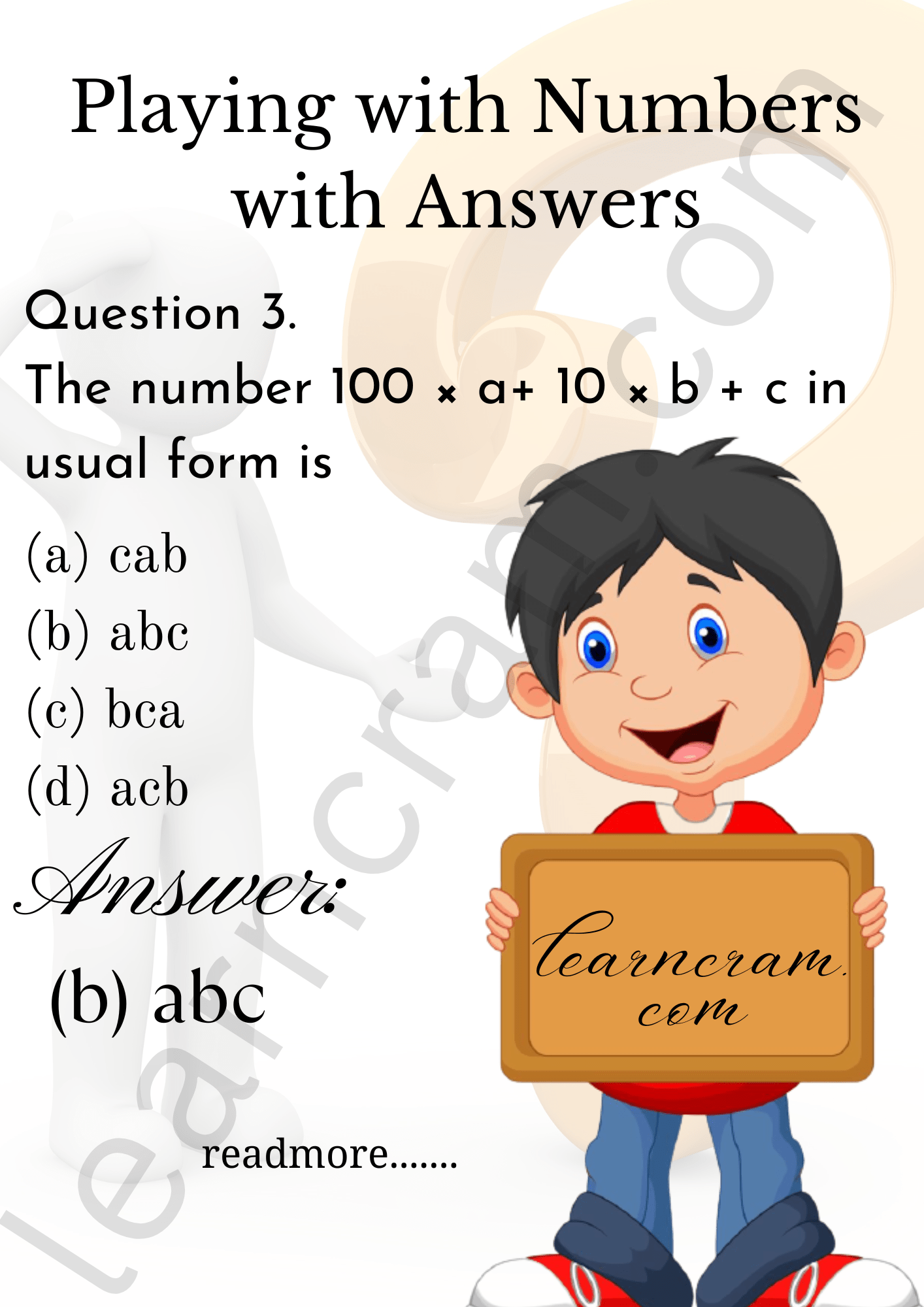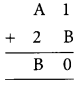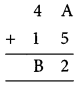# MCQ Questions for Class 8 Maths Chapter 16 Playing with Numbers with Answers

We have compiled the NCERT MCQ Questions for Class 8 Maths Chapter 16 Playing with Numbers with Answers Pdf free download covering the entire syllabus. Practice MCQ Questions for Class 8 Maths with Answers on a daily basis and score well in exams. Refer to the Playing with Numbers Class 8 MCQs Questions with Answers here along with a detailed explanation.

## Playing with Numbers Class 8 MCQs Questions with Answers

Choose the correct option.

Question 1.
Ifwhere z and y are single – digit numbers such that z – y = 3 then the value of y and z are
(a) 3, 6
(b) 6, 9
(c) 5, 4
(d) 4, 5

Question 2.
The generalised form of the number 123 is …………..
(a) 3 × 100 + 1 × 10 + 2
(b) 2 × 100 + 3 × 10 + 1
(c) 1 × 100 + 2 × 10 + 3
(d) None of these

Answer: (c) 1 × 100 + 2 × 10 + 3Question 3.
The number 100 × a+ 10 × b + c in usual form is
(a) cab
(b) abc
(c) bca
(d) acbQuestion 4.
Find the value of A, B in the following:(a) 6, 9
(b) 6, 6
(c) 9, 6
(d) 9, 9

Question 5.
If the number 1 × 8 is divisible by 3 then x is equal to
(a) 4
(b) 5
(c) 7
(d) 6

Question 6.
If the three-digit number x27 is divisible by 9, then the value of x is
(a) 8
(b) 7
(c) 6
(d) 9

Question 7.
Which of the following number is not divisible by 3?
(a) 123
(b) 132
(c) 134
(d) 231

Question 8.
The number 28221 is divisible by which of the following?
(a) 2
(b) 3
(c) 6
(d) 9

Question 9.
If a number is divisible by 5 then which of the following can be its one’s digit?
(a) 2
(b) 3
(c) 4
(d) 5

Question 10.
If the number 9y7 is a multiple of 3 then y =
(a) 4
(b) 3
(c) 6
(d) 2 or 5 or 8

Answer: (d) 2 or 5 or 8

Question 11.
If M is a number such that M ÷ 5 gives a remainder of 1, then which of the following is the ones-digit of M?
(a) 1
(b) 6
(c) 1 or 6
(d) none of these

Question 12.
A number divisible by 9 is also divisible by:
(a) 3
(b) 6
(c) 11
(d) none of these.

Fill in the blanks

Question 1.
If 10 a+ b is a ………………… digit number where (a ≠ 0) and b are single digit whole numbers.

Question 2.
If 49032 is divisible by 24, then it is divisible by 3 and …………………

Question 3.
The usual form of the number 9 × 100 + 7 × 1 is …………………